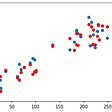# All about Support Vector Machine

1. Introduction:

SVM is a supervised Machine learning model which is used for both Classification (Support Vector Classifier SVC) and Regression (Support Vector Regression SVR) tasks. However, it is most often used for classification.

The goal of the Support Vector Classifier (SVC) is to find a hyperplane (in n-dimensional space) that separates the positive (+ve) and negative (-ve) classes (in case of binary classification) as away as possible such a hyperplane is called Margin Maximizing hyperplane.

2. Margin Maximizing Hyperplane:

Let’s understand how to find Margin Maximizing Hyperplane?

To separate the two classes of data points, there are many possible hyperplanes that could be selected. Our objective is to find a plane that has the maximum margin, i.e. the maximum distance between data points of both classes. Maximizing the margin distance provides some reinforcement so that future data points can be classified with more confidence.

π+: hyperplane parallel to π (optimal hyperplane) in +ve class side which touches the first +ve point (called support vector).

π-: hyperplane parallel to π (optimal hyperplane) in the -ve class side, which touches the first -ve point (called support vector).

Support vectors are the data points on π+ and π-, that influence the position and orientation of the optimal hyperplane.

Margin is the distance between π+ and π-.

3. Why Margin Maximizing Hyperplanes:

The maximum margin is important because the larger the margin better will be the classification and misclassification will be lesser so the generalization error. In logistic regression, we take the output of the linear function and squash the value within the range of [0, 1] using the sigmoid function. If the squashed value is greater than a threshold value(0.5) we assign it a label 1, else we assign it a label 0. In SVM, we take the output of the linear function and if that output is greater than or equal to 1, we identify it with one class and if the output is -1, we identify it with another class. Since the threshold values are changed to 1 and -1 in SVC, we obtain this reinforcement range of values [-1,1] which acts as margin.

4. Mathematics behind the scene

Now our task is to find the distance (d)between 2 parallel hyperplanes and maximize that distance.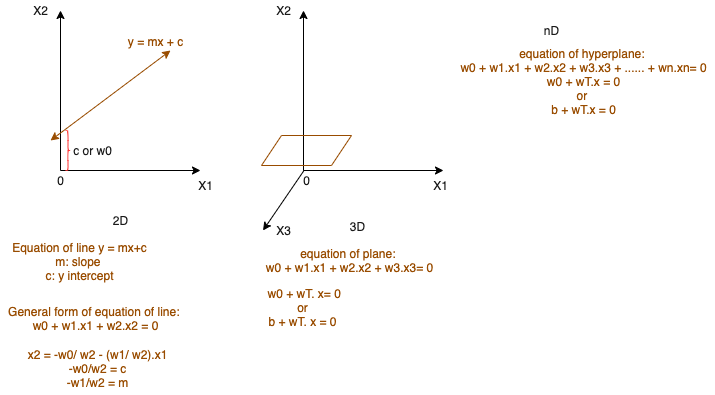Equation of line (in 2-dimension), plane (in 3-dimension) and hyperplane (in n-dimension)

Below are the equation of hyperplanes π, π+ and π-:

wT x + b = 0: hyperplane passing through the origin, w is a normal vector perpendicular to hyperplane and b is the intercept

wT x + b = 1: hyperplane in positive direction or π+

wT x + b = -1: hyperplane in negative direction or π-

Distance between 2 parallel hyperplanes π+: wT x + b =1 and π-: wT x + b = -1 using above formula would be: D1=-1, D2=1, A = w0, B=w1, C=w2

||w|| is l2 norm of w or euclidean distance.

Now we need to maximize margin (d) with the below constraints:

For correctly classified data points, the actual class (yi) and predicted class (wT.x + b) would be of the same sign, either both are positive or both are negative. And the product of which (signed distance: yi . (wT. x + b)) would always be positive (≥ 1).

For incorrectly classified data points, the actual class (yi) and predicted class (wT.x + b) would be of different signs, one is positive, and the other is negative or vice versa. And the product of which (signed distance: yi . (wT. x + b)) would always be negative (≤ 1).

5. Hard Margin SVM

In summary, below is the optimization problem of SVM with Hard Margin, where no outliers are allowed in the margin and data is linearly separable:

6. Soft Margin SVM and Hinge Loss

But what if data is non-linearly separable, we can use Soft Margin SVM. In this scenario, we allow a few misclassifications to happen. So we need to minimize the misclassification error, which means that we have to deal with one more constraint. Second, to minimize the error, we should define a loss function. A common loss function used for soft margin is hinge loss.

Hinge Loss is 0 for correctly classified data points, for incorrectly classified points hinge loss will be determined by how far it is from the correct hyperplane.

7. Hyperparameter- C (or slack variable):

The regularization parameter C is used only in Soft Margin SVM, which is called a slack penalty. C adds a penalty for each misclassified data point. Typically, the value of C should be [0.1, 100], the best value can be identified using Cross-Validation. In summary:

C HIGHER → Penalty to misclassified points LARGER → margin LOWER → misclassified points inside margin LOWEROVERFIT

C LOWER → penalty to misclassified points LOWER → margin LARGER→ misclassified points inside margin LARGERUNDER FIT

8. Kernel Function:

What if we can’t find the threshold for non-linearly separable data. For example, the amount of dosage should not be more or lower than a certain value. The solution is to use SVM:

2. Move the data into a higher dimension (for example, create y-axis coordinate as x² in case of 1-dimension non-linearly separable data). This task is performed using the Kernel function (Radial Basis Kernel or Polynomial Kernel) to systematically find Support Vector Classifiers in higher dimensions.

Kernel functions only calculate the relationships between every pair of points as if they are in the higher dimensions, they don’t actually do the transformation.

3. Find a Support Vector Classifier that separates the higher dimensional data into 2 groups.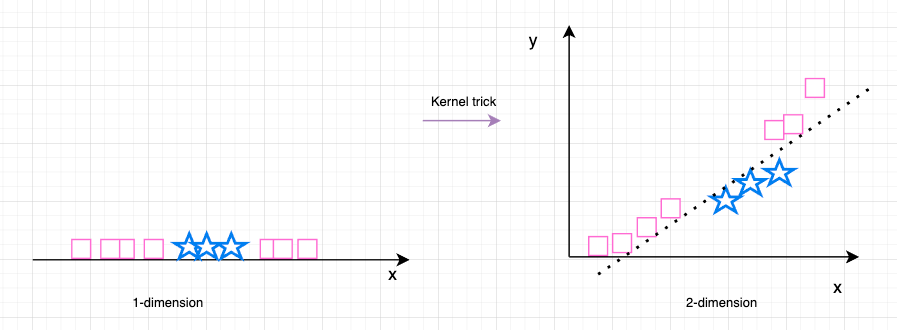Kernel trick to move 1d (non-linear data) to 2d (linearly separable data)

9. RBF kernel and associated hyperparameter- γ (or Gamma):

RBF Kernel finds Support vector classifiers in infinite dimensions, it behaves similarly to weighed Nearest Neighbour. RBF kernel function K is given by the below formula:

Gamma γ is hyperparameter only in the case of the RBF kernel, which is inversely proportional to standard deviation σ. As γ increases, the region of similarity increases. Typically, the γ value is between [0.0001, 10], the best value can be identified using Cross-Validation.

γ HIGHER → σ LOWER → normal curve THINNER/ peaked → similar #points groups together LOWER → region of similarity LOWER → region of dissimilarity HIGHEROVERFIT

γ LOWER → σ HIGHER → normal curve THICKER/ broader → similar #points groups together HIGHER → region of similarity HIGHER → region of dissimilarity LOWERUNDER FIT

10. Python code example with the linear kernel:

Here is an example of SVC with python code. To see support vector machines in action, I’ve generated a random dataset with different ratios of +ve, -ve classes: (100, 2), (100, 20), (100, 40), (100, 80). Here’s the code snippet that generates and plots the data:

Here is the output of the scatter plots:

Now we try to fit the SVC model with different regularization parameters C {0.001, 1, 100} and draw a margin maximizing hyperplane. I also draw the π+ and π- and highlighted the support vectors in each plot.

Here we can observe that as C increases, the margin reduced.

Git link for full code: https://github.com/heena-sharma-sys/Machine-Learning/blob/main/SVMTest.ipynb

11. References:

--

--

--

## More from Heena Sharma

Love podcasts or audiobooks? Learn on the go with our new app.

## My Recommendations to Learn Machine Learning in Production## Automatic MissingValuesHandler library with a Random Forest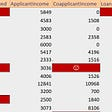## Auxiliary Loss Optimization for Hypothesis Augmentation for DGA Domain Detection## An Introduction to Naïve Bayes Classifier## Long Short Term Memory networks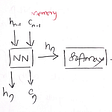## Training ML models directly from GitHub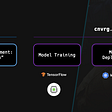## Random forests for variable importance 🌲🌳🎄## ReLU Activation Function## Naive Bayes in Machine Learning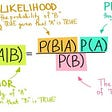## Machine Learning — Week 1